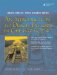const changes the signature of a member function. This means that functions can be overloaded on const-ness. Example 5.16 shows a homemade vector class with member functions overloaded in this way.

 ```#ifndef CONSTOVERLOAD_H #define CONSTOVERLOAD_H #include class Point3 { <-- 1 public: friend std::ostream& operator<<(std::ostream& out, const Point3& v); Point3(double x = 0, double y = 0, double z = 0); double& operator[](int index); const double& operator[](int index) const; <-- 2 Point3 operator+(const Point3& v) const; Point3 operator-(const Point3& v) const; Point3 operator*(double s) const; <-- 3 private: static const int cm_Dim = 3; double m_Coord[cm_Dim]; }; #endif ```   (1)a 3D point (of double) (2)overloaded on const-ness (3)scalar multiplication

 1 In Example 5.17, the compiler can tell the difference between calls to the const and to the non-const versions of operator[] based on the const-ness of the object.

 ```#include "constoverload.h" #include int main( ) { using namespace std; Point3 pt1(1.2, 3.4, 5.6); const Point3 pt2(7.8, 9.1, 6.4); double d ; d = pt2; <-- 1 cout << d << endl; d = pt1; <-- 2 cout << d << endl; d = pt1; <-- 3 cout << d << endl; pt1 = 8.7; <-- 4 cout << pt1 << endl; // pt2 = 'd'; cout << pt2 << endl; return 0; } ```   (1)__________ (2)__________ (3)__________ (4)__________
1. Which operator is called for each of the notes?
2. Why is the last assignment commented out?
3. The operator function definitions are shown in Example 5.18. The fact that the two function bodies are identical is worth pondering. If index is in range, each function returns m_Coord[index], so what is the difference between them?

 ```[ . . . . ] const double& Point3::operator[](int index) const { if ((index >= 0) && (index < cm_Dim)) return m_Coord[index]; else return zero(index); } double& Point3::operator[](int index) { if ((index >= 0) && (index < cm_Dim)) return m_Coord[index]; else return zero(index); } [ . . . . ] ```

### Inline FunctionsAn Introduction to Design Patterns in C++ with Qt 4
ISBN: 0131879057
EAN: 2147483647
Year: 2004
Pages: 268